# Chamber

(diff) ← Older revision | Latest revision (diff) | Newer revision → (diff)

in a finite-dimensional real affine space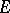, with respect to a locally finite set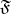of hyperplanes inA connected component of the set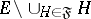. A chamber is an open convex subset of.

Letbe a set of hyperplanes insuch that the groupof motions ofgenerated by the orthogonal reflections with respect to the hyperplanes ofis a discrete group of transformations of, and such that moreover the systemis invariant with respect to. In this case one speaks about a chamber relative to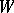. The groupacts simply transitively on the set of all chambers and is generated by the setof orthogonal reflections with respect to hyperplanes ofcontaining the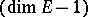-dimensional faces of any fixed chamber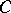; moreover, the pair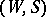is a Coxeter system and the closure ofis a fundamental domain of. The structure of(the description of the dihedral angles between the walls) completely determines the structure ofas an abstract group. The study of this structure is an important step in obtaining a complete classification of the discrete groups generated by the reflections in(see Coxeter group). Along with this classification, a complete description is obtained of the structure of chambers for such groups.

Ifis the Weyl group of a root system of a semi-simple Lie algebra, a chamber relative tois called a Weyl chamber of.

The notion of a chamber can also be defined for hyperplanes and discrete groups generated by reflections in Lobachevskii space or on a sphere .

How to Cite This Entry:
Chamber. Encyclopedia of Mathematics. URL: http://encyclopediaofmath.org/index.php?title=Chamber&oldid=12004
This article was adapted from an original article by V.L. Popov (originator), which appeared in Encyclopedia of Mathematics - ISBN 1402006098. See original article